# 坐标系描述

A p B o ^Ap_{Bo} 表示在参考坐标系 { A } \{A\} 下坐标系 { B } \{B\} 的原点坐标，并用旋转矩阵 B A R ^A_BR 描述坐标系 { B } \{B\} 在参考坐标系 { A } \{A\} 下的姿态，则坐标系 { B } \{B\} 的姿态可表示为：
{ B } = { A p B o B A R } \{B\}=\{\enspace^Ap_{Bo}\quad{}^A_BR\enspace\}

## 平移映射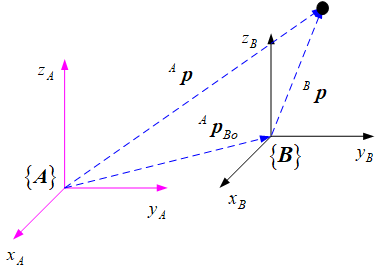A p B o ^Ap_{Bo} 为坐标系 { B } \{B\} 相对于 { A } \{A\} 的平移矢量，若点 p p 在坐标系 { B } \{B\} 下的位置为 B p ^Bp ，则它相对于坐标系 { A } \{A\} 的位置 A p ^Ap 可表示为：
A p = B p + A p B o {}^Ap={}^Bp+{}^Ap_{Bo}

## 旋转映射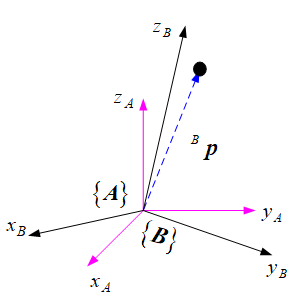B A R {}^A_BR 为坐标系 { B } \{B\} 相对于 { A } \{A\} 的方位，若点 p p 在坐标系 { B } \{B\} 下的位置为 B p ^Bp ，则它相对于坐标系 { A } \{A\} 的位置 A p ^Ap 可表示为：
A p = B A R   B p {}^Ap={}^A_BR\:{}^Bp

A B R = B A R − 1 = B A R T {}^B_AR={}^A_BR^{-1}={}^A_BR^T

## 一般刚体变换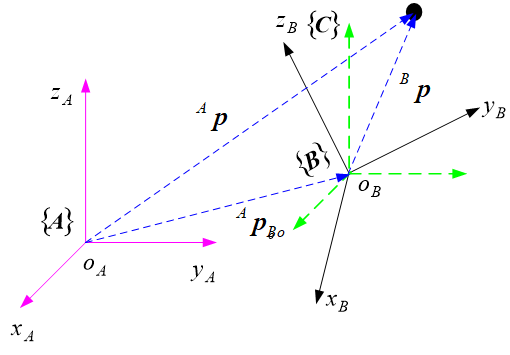A p = B A R   B p + A p B o {}^Ap={}^A_BR\:{}^Bp+{}^Ap_{Bo}

[ A p 1 ] = [ B A R A p B o 0 1 ] [ B p 1 ] = B A T   B p \begin{bmatrix}{}^Ap\\1\end{bmatrix}=\begin{bmatrix}{}^A_BR&{}^Ap_{Bo}\\0&1\end{bmatrix}\begin{bmatrix}{}^Bp\\1\end{bmatrix}={}^A_BT\:{}^Bp

B A T = [ B A R A p B o 0 1 ] {}^A_BT=\begin{bmatrix}{}^A_BR&{}^Ap_{Bo}\\0&1\end{bmatrix}

## 齐次坐标

p = [ x y z 1 ] = [ ω x ω y ω z ω ] p=\begin{bmatrix}x\\y\\z\\1\end{bmatrix}=\begin{bmatrix}\omega x\\\omega y\\\omega z\\\omega\end{bmatrix}

X 轴 : [ 1 0 0 0 ] Y 轴 : [ 0 1 0 0 ] Z 轴 : [ 0 0 1 0 ] 任 意 无 穷 远 处 : [ a b c 0 ] X轴:\begin{bmatrix}1\\0\\0\\0\end{bmatrix}\quad Y轴:\begin{bmatrix}0\\1\\0\\0\end{bmatrix}\quad Z轴:\begin{bmatrix}0\\0\\1\\0\end{bmatrix}\quad 任意无穷远处: \begin{bmatrix}a\\b\\c\\0\end{bmatrix}

# 变换矩阵运算与刚体群

## 运动算子

B A T = [ B A R A p B o 0 1 ] = [ I 3 × 3 A p B o 0 1 ] [ B A R ( k , θ ) 0 0 1 ] = T r a n s ( A p B o ) R o t ( k , θ ) \begin{aligned} {}^A_BT =&\begin{bmatrix}{}^A_BR&{}^Ap_{Bo}\\0&1\end{bmatrix}\\ =&\begin{bmatrix}I_{3\times3}&{}^Ap_{Bo}\\0&1\end{bmatrix}\begin{bmatrix}{}^A_BR(k,\theta)&0\\0&1\end{bmatrix}\\ =&Trans({}^Ap_{Bo})Rot(k,\theta) \end{aligned}
T r a n s ( A p B o ) Trans({}^Ap_{Bo}) 表示沿着位移矢量 A p B o {}^Ap_{Bo} 进行平移， R o t ( k , θ ) Rot(k,\theta) 则表示绕着过原点的轴 k k 旋转 θ \theta 角。

### 平移算子

A p 2 = A p 1 + A p {}^Ap_2={}^Ap_1+{}^Ap

A p 2 = T r a n s ( A p )   A p 1 {}^Ap_2=Trans({}^Ap)\:{}^Ap_1

### 旋转算子

A p 2 = R   A p 1 {}^Ap_2=R\:{}^Ap_1

A p 2 = R o t ( k , θ )   A p 1 {}^Ap_2=Rot(k,\theta)\:{}^Ap_1

### 运动算子一般形式

A p ( t ) = T ( t )   A p ( 0 ) {}^Ap(t)=T(t)\:{}^Ap(0)

## 变换矩阵的运算

### 变换矩阵乘法

C A T = B A T   C B T = [ B A R   C B R B A R   B p C o + A p B o 0 1 ] {}^A_CT={}^A_BT\:{}^B_CT=\begin{bmatrix}{}^A_BR\:{}^B_CR&{}^A_BR\:{}^Bp_{Co}+{}^Ap_{Bo}\\0&1\end{bmatrix}

• 运动相对固定坐标系而言：满足左乘规则，即变换顺序从右至左
• 运动相对运动坐标系而言：满足右乘规则，即变换顺序从左至右
• 变换的顺序不能调换进行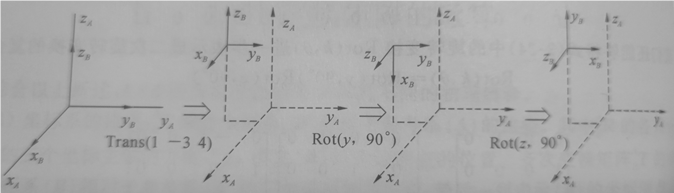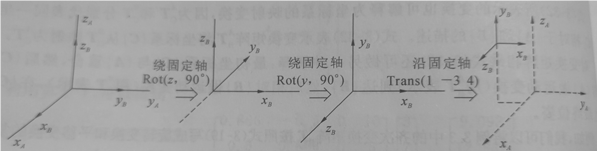### 变换矩阵求逆

A B R = B A R − 1 = B A R T {}^B_AR={}^A_BR^{-1}={}^A_BR^T

B ( A p B o ) = A B R   A p B o + B p A o {}^B({}^Ap_{Bo})={}^B_AR\:{}^Ap_{Bo}+{}^Bp_{Ao}

B p A o = − A B R   A p B o = − B A R T   A p B o {}^Bp_{Ao}=-{}^B_AR\:{}^Ap_{Bo}=-{}^A_BR^T\:{}^Ap_{Bo}

A B T = [ B A R T − B A R T   A p B o 0 1 ] {}^B_AT=\begin{bmatrix}{}^A_BR^T&-{}^A_BR^T\:{}^Ap_{Bo}\\0&1\end{bmatrix}

## 刚体变换群

S E ( 3 ) = { T = [ R t 0 T 1 ] ∈ ℜ 4 × 4 ∣ R ∈ S O ( 3 ) , t ∈ ℜ 3 } = ℜ 3 × S O ( 3 ) SE(3)=\bigl\{T=\begin{bmatrix}R&t\\0^T&1\end{bmatrix}\in \Re^{4\times4}\big\vert R\in SO(3),t\in\Re^{3}\bigr\}=\Re^{3}\times SO(3)
S E ( 3 ) SE(3) 又称为三维空间的特殊欧式群（Special Euclidean Group ），推广到n维空间中可得：
S E ( n ) = { T = [ R t 0 T 1 ] ∈ ℜ n + 1 × n + 1 ∣ R ∈ S O ( n ) , t ∈ ℜ n } = ℜ n × S O ( n ) SE(n)=\bigl\{T=\begin{bmatrix}R&t\\0^T&1\end{bmatrix}\in \Re^{n+1\times n+1}\big\vert R\in SO(n),t\in\Re^{n}\bigr\}=\Re^{n}\times SO(n)
S E ( n ) SE(n) 为李群，当n=2时， S E ( 2 ) SE(2) 表示刚体的平面运动，其单位元为 I 3 I_3 ；当n=3时， S E ( 3 ) SE(3) 表示刚体

### Java多线程并发面试问答_淡定的蜗牛的博客-程序员秘密

Java并发面试问答什么是原子操作？Java并发API中的原子类是什么？原子操作在单个任务单元中执行，而不受其他操作的干扰。在多线程环境中，原子操作是必需的，以避免数据不一致。int++不是原子操作。因此，当一个线程读取其值并将其递增1时，另一线程读取了较早的值，从而导致错误的结果。为了解决这个问题，我们必须确保对count的增量操作是原子的，我们可以使用Synchronization做到...

### Compilation Error 解决方案汇集 _compilation error怎么解决_德仔的博客-程序员秘密

<br />次遇见Compilation Error ,这次可就有办法对付了. 当然,这也算是一个BUG啦<br /><br /><br /><br />来源:http://www.dezai.cn/Blog/article.asp?id=386<br /><br />方案一:<br /><br />如果是在开发状态下编译出现Compilation <br />Error的话<br />(1)清理解决方案 Clean the <br />solution<br />(2)根据所报的错误提示,查找对应的页面划

### 量子学习及思考7-量子基本数学知识_aibo110110的博客-程序员秘密

NM的才刚入门就是一堆数学知识,可见数学才是一切科学的本源.所谓狗屁科学,只不过是数学的一个实例或者是一个近似的表达而已.越接近数学的,离正确性越近. 本人数学基础太垃圾,好在现在有互联网,本人说过,程序员+互联网=超人,有说过吗?有,只不过现在明确提出这个超人定理: 超人定理:超人=程序员+互联网 我们再看看其它的算法: 计算机系统=软件...

### hql语句大全_hql由哪些句子组成_kkrgwbj的博客-程序员秘密

Hibernate配备了一种非常强大的查询语言，这种语言看上去很像SQL。但是不要被语法结构 上的相似所迷惑，HQL是非常有意识的被设计为完全面向对象的查询，它可以理解如继承、多态 和关联之类的概念。  第 15 章 HQL: Hibernate查询语言 Hibernate配备了一种非常强大的查询语言，这种语言看上去很像SQL。但是不要被语法结构 上的相似所迷惑，HQL是非常有意识